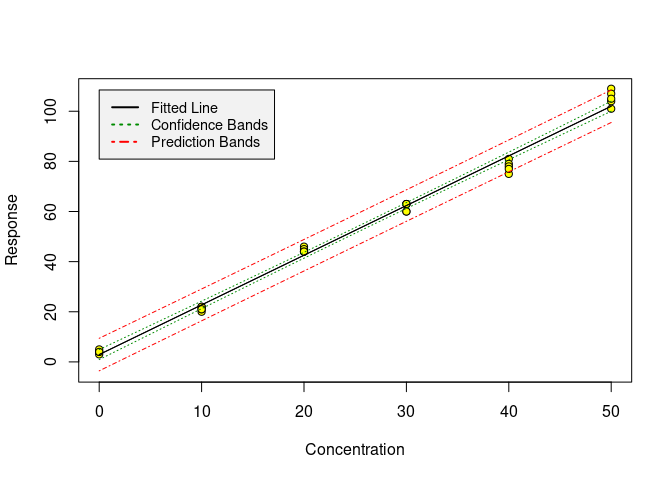# chemCal - Calibration functions for analytical chemistry

## Overview

chemCal is an R package providing some basic functions for conveniently working with linear calibration curves with one explanatory variable.

## Installation

From within R, get the official chemCal release using

``install.packages("chemCal")``

## Usage

chemCal works with univariate linear models of class `lm`. Working with one of the datasets coming with chemCal, we can produce a calibration plot using the `calplot` function:

### Plotting a calibration

``````library(chemCal)
m0 <- lm(y ~ x, data = massart97ex3)
calplot(m0)``````### LOD and LOQ

If you use unweighted regression, as in the above example, we can calculate a Limit Of Detection (LOD) from the calibration data.

``````lod(m0)
#> \$x
#>  5.407085
#>
#> \$y
#>  13.63911``````

This is the minimum detectable value (German: Erfassungsgrenze), i.e. the value where the probability that the signal is not detected although the analyte is present is below a specified error tolerance beta (default is 0.05 following the IUPAC recommendation).

You can also calculate the decision limit (German: Nachweisgrenze), i.e. the value that is significantly different from the blank signal with an error tolerance alpha (default is 0.05, again following IUPAC recommendations) by setting beta to 0.5.

``````lod(m0, beta = 0.5)
#> \$x
#>  2.720388
#>
#> \$y
#>  8.314841``````

Furthermore, you can calculate the Limit Of Quantification (LOQ), being defined as the value where the relative error of the quantification given the calibration model reaches a prespecified value (default is 1/3).

``````loq(m0)
#> \$x
#>  9.627349
#>
#> \$y
#>  22.00246``````

### Confidence intervals for measured values

Finally, you can get a confidence interval for the values measured using the calibration curve, i.e. for the inverse predictions using the function `inverse.predict`.

``````inverse.predict(m0, 90)
#> \$Prediction
#>  43.93983
#>
#> \$`Standard Error`
#>  1.576985
#>
#> \$Confidence
#>  3.230307
#>
#> \$`Confidence Limits`
#>  40.70952 47.17014``````

If you have replicate measurements of the same sample, you can also give a vector of numbers.

``````inverse.predict(m0, c(91, 89, 87, 93, 90))
#> \$Prediction
#>  43.93983
#>
#> \$`Standard Error`
#>  0.796884
#>
#> \$Confidence
#>  1.632343
#>
#> \$`Confidence Limits`
#>  42.30749 45.57217``````

## Reference

You can use the R help system to view documentation, or you can have a look at the online documentation.